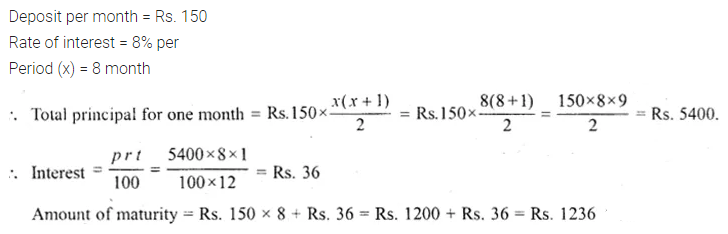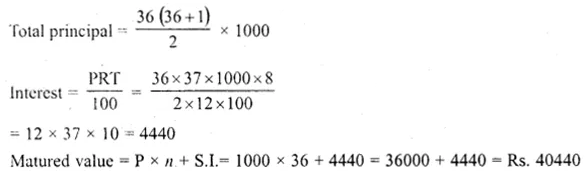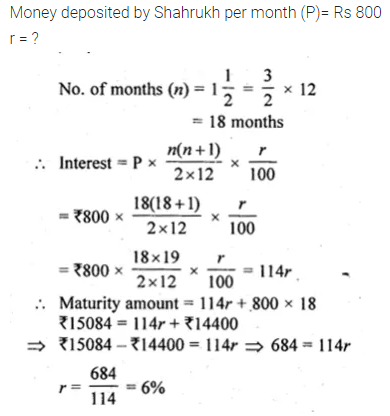# ML Aggarwal Class 10 Solutions for ICSE Maths Chapter 2 Banking Ex 2

## ML Aggarwal Class 10 Solutions for ICSE Maths Chapter 2 Banking Ex 2

Question 1.
Shweta deposits Rs. 350 per month in a recurring deposit account for one year at the rate of 8% p.a. Find the amount she will receive at the time of maturity.
Solution:Question 2.
Salom deposited Rs 150 per month in a bank for 8 months under the Recurring Deposit Scheme. ‘What will be the maturity value of his deposit if the rate of interest is 8% per annum?
Solution:Question 3.
Mrs Goswami deposits Rs. 1000 every month in a recurring deposit account for 3 years at 8% interest per annum. Find the matured value. (2009)
Solution:Question 4.
Kiran deposited Rs. 200 per month for 36 months in a bank’s recurring deposit account. If the banks pay interest at the rate of 11% per annum, find the amount she gets on maturity?
Solution:Question 5.
Haneef has a cumulative bank account and deposits Rs. 600 per month for a period of 4 years. If he gets Rs. 5880 as interest at the time of maturity, find the rate of interest.
Solution:Question 6.
David opened a Recurring Deposit Account in a bank and deposited Rs. 300 per month for two years. If he received Rs. 7725 at the time of maturity, find the rate of interest per annum. (2008)
Solution:Question 7.
Mr Gupta-opened a recurring deposit account in a bank. He deposited Rs. 2500 per month for two years. At the time of maturity, he got Rs. 67500. Find :
(i) the total interest earned by Mr Gupta.
(ii) the rate of interest per annum.
Solution:Question 8.
Shahrukh opened a Recurring Deposit Account in a bank and deposited Rs 800 per month for $$1 \frac { 1 }{ 2 }$$ years. If he received Rs 15084 at the time of maturity, find the rate of interest per annum.
Solution:Question 9.
Mohan has a recurring deposit account in a bank for 2 years at 6% p.a. simple interest. If he gets Rs 1200 as interest at the time of maturity, find:
(i) the monthly instalment
(ii) the amount of maturity. (2016)
Solution:Question 10.
Mr R.K. Nair gets Rs 6,455 at the end of one year at the rate of 14% per annum in a recurring deposit account. Find the monthly instalment.
Solution:Question 11.
Samita has a recurring deposit account in a bank of Rs 2000 per month at the rate of 10% p.a. If she gets Rs 83100 at the time of maturity. Find the total time for which the account was held.
Solution: